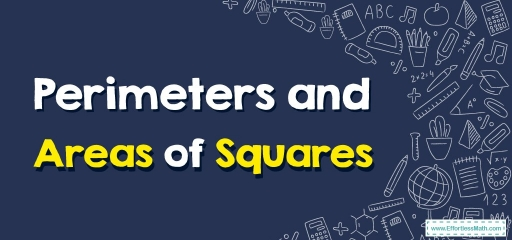# Perimeters and Areas of Squares

Want to know more about how to get the perimeter and area of a square? So stay tuned for the rest of this article.Squares are closed $$2$$-dimensional shapes ($$2D$$ shapes) having $$4$$ sides. All $$4$$ sides of a square are identical as well as parallel to one another.

## Definition of a Square

• The opposing sides are parallel.
• All 4 sides are identical.
• All angles equal $$90°$$.

A few of the vital characteristics of squares are shown here:

• Squares are quadrilaterals having four sides as well as four vertices.
• A square’s $$4$$ sides are equivalent to one another.
• A square’s opposite sides are parallel to one another.
• A square’s interior angle at each vertex equals $$90°$$.
• The total of all inner angles equals $$360°$$.
• A  square’s diagonals bisect one another at $$90°$$.
• The diagonals’ length is equivalent.
• Because the square’s sides are parallel, it’s additionally known as a parallelogram.
• The diagonals’ length in a square is larger than the sides.
• The diagonals split up the square into $$2$$ congruent triangles.

## Square’s area

A square’s area is the space it occupies. The formula to get a square’s area is stated as, Area of square $$= s$$2; where ‘s’ is the square’s side. It’s stated in square units such as $$cm$$2, $$m$$2, etc.

## Square’s perimeter

A square’s perimeter is the complete boundary length. Thus, the square’s perimeter can be determined by adding the length of all the sides. Because squares have $$4$$ four sides, you have to add all $$4$$ sides of them to locate their perimeter.

You can utilize the formula of the perimeter of a square to locate the boundary length. A perimeter of a square $$= side + side + side + side$$. Consequently, $$Perimeter of Square = (4 × Side)$$. It’s shown via linear units such as $$cm, m$$, inches, etc.

### Perimeters and Areas of Squares –Example 1:

Find perimeter and area of square.

A:____,B:____

Solution:

The Area of square $$= side^2 →$$ Area of square $$= 25^2 =625$$.

The perimeter of Square $$= (4 × Side )→$$ Perimeter of Square $$=4 × 25=100$$

### Perimeters and Areas of Squares –Example 2:

Find the area and perimeter of the square with a side of $$12$$.

Solution:

The Area of square $$= side^2 →$$ Area of square $$= 12^2 =144$$.

The perimeter of Square $$= (4 × Side )→$$ Perimeter of Square $$=4 × 12=48$$

## Exercises for Perimeters and Areas of Squares

Find perimeter and area of squares.

1)

2)

1. $$\color{blue}{A:225,P:60}$$
2. $$\color{blue}{A:324,P:72}$$

### What people say about "Perimeters and Areas of Squares - Effortless Math: We Help Students Learn to LOVE Mathematics"?

No one replied yet.

X
30% OFF

Limited time only!

Save Over 30%

SAVE $5 It was$16.99 now it is \$11.99